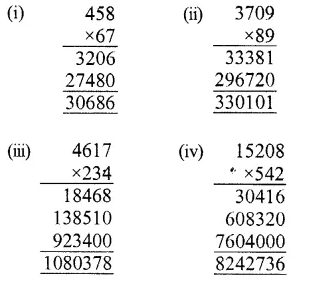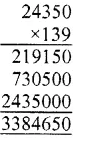## RS Aggarwal Class 6 Solutions Chapter 3 Whole Numbers Ex 3D

These Solutions are part of RS Aggarwal Solutions Class 6. Here we have given RS Aggarwal Solutions Class 6 Chapter 3 Whole Numbers Ex 3D.

Other Exercises

Question 1.
Solution:
(i) 246 x 1 = 246
(By multiplicative property of 1)
(ii) 1369 x 0 = 0
(By multiplicative property of 0)
(iii) 593 x 188 = 188 x 593
(By commutative law of multiplication)
(iv) 286 x 753 = 753 x 286
(By commutative law of multiplication)
(v) 38 x (91 x 37) = 91 x (38 x 37)
(By associative law of multiplication)
(vi) 13 x 100 x 1000 = 1300000
(vii) 59 x 66 + 59 x 34 = 59 x (66 + 34)
(By distributive law of multiplication)
(vii) 68 x 95 = 68 x 100 – 68 x 5

Question 2.
Solution:
(i) Commutative law of multiplication
(ii) Closure property
(iii) Associative law of multiplication
(iv) Multiplicative property of 1
(v) Multiplicative property of 0
(vi) Distributive law of multiplication over addition in whole numbers
(vii) Distributive law of multiplication over subtraction in whole numbers

Question 3.
Solution:
Using the law of distribution over addition and subtraction
(i) 647 x 13 + 647 x 7
= 647 x (13 + 7)
= 647 x 20
= 12940
(ii) 8759 x 94 + 8759 x 6
= 8759 x (94 + 6)
= 8759 x 100
= 875900
(iii) 7459 x 999 + 7459
= 7459 x 999 + 7459 x 1
= 7459 x (999 + 1)
= 7459 x 1000
= 7459000
(iv) 9870 x 561 – 9870 x 461
= 9870 x (561 – 461)
= 9870 x 100
= 987000
(v) 569 x 17 + 569 x 13 + 569 x 70
= 569 x (17 + 13 + 70)
= 569 x 100
= 56900
(vi) 16825 x 16825 – 16825 x 6825
= 16825 x (16825 – 6825)
= 16825 x 10000
= 168250000

Question 4.
Solution:
(i) 2 x 1658 x 50 = 1658 x (2 x 50) (Associative law of multiplication)
= 1658 x 100
= 165800
(ii) 4 x 927 x 25 = 927 x (4 x 25) (Associative law of multiplication)
= 927 x 100
= 92700
(iii) 625 x 20 x 8 x 50
(By associative law of multiplication)
(625 x 8) x (20 x 50)
= 5000 x 1000
= 5000000
(iv) 574 x (625 x 16)
= 574 x 10000
= 5740000
(v) 250 x 60 x 50 x 8
= (250 x 8) x (60 x 50)
(By associative law)
= 2000 x 3000
= 6000000
(vi) 8 x 125 x 40 x 25
= (8 x 125) x (40 x 25)
= 1000 x 1000
= 1000000

Question 5.
Solution:
Using distributive law of multiplication over addition or subtraction,
(i) 740 x 105
= 740 x (100 + 5)
= 740 x 100 + 740 x 5
= 74000 + 3700
= 77700
(ii) 245 x 1008
= 245 x (1000 + 8)
= 245 x 1000 + 245 x 8
= 245000 + 1960
= 246960
(iii) 947 x 96
= 947 x (100 – 4)
= 947 x 100 – 947 x 4
= 94700 – 3788
= 90912
(iv) 996 x 367
= 367 x (1000 – 4)
= 367 x 1000 – 367 x 4
= 367000 – 1468
= 365532
(v) 472 x 1097
= 472 x (1100 – 3)
= 472 x 1100 – 472 x 3
= 519200 – 1416
= 517784
(vi) 580 x 64
= 580 x (60 + 4)
= 580 x 60 + 580 x 4
= 34800 + 2320
= 37120
(vii) 439 x 997
= 437 x (1000 – 3)
= 439 x 1000 – 439 x 3
= 439000 – 1317
= 437683
(viii) 1553 x 198
= 1553 x (200 – 2)
= 1553 x 200 – 1553 x 2
= 310600 – 3106
= 307494

Question 6.
Solution:
(i) 3576 x 9 = 3576 x (10 – 1)
= 3576 x 10 – 3576 x 1
= 35760 – 3576
= 32184
(ii) 847 x 99 = 84 x (100 – 1)
= 847 x 100 – 847 x 1
= 84700 – 847
= 83853
(iii) 2437 x 999 = 2437 x (1000 – 1)
= 2437 x 1000 – 2437 x 1
= 2437000 – 2437
= 2434563

Question 7.
Solution:Question 8.
Solution:
Largest 3-digit number = 999
Largest 5-digit number = 99999
Required product = 99999 x 999
= 99999 x (1000 – 1)
= 99999 x 1000-99999 x 1
= 99999000 – 99999
= 9,98,99,001

Question 9.
Solution:
Speed of car = 75 km per hour
In 1 hour, distance covered by a car = 75 km
.’. In 98 hours, distance will be covered = 75 x 98
= 75 x (100 – 2)
= 75 x 100 – 75 x 2
= 7500 – 150
= 7350 km

Question 10.
Solution:
Cost of 1 set of VCR = Rs. 24350
Cost of 139 sets of VCR = Rs. 24350 x 139
= Rs. 3384650Question 11.
Solution:
Cost of 1 house = Rs. 450000
Cost of 197 houses = Rs. 450000 x 197
= Rs. 450000 x (200 – 3)
= Rs. (450000 x 200 – 450000 x 3)
= Rs. (90000000 – 1350000)
= Rs. 88650000

Question 12.
Solution:
Cost of each chair = Rs. 1065
Cost of 50 chairs = Rs. 1065 x 50
= Rs. 53250
Cost of each blackboard = Rs. 1645
Cost of 30 blackboards = Rs. 1645 x 30
= Rs. 49350
Total cost of 50 chairs and 30 blackboards = Rs. 53250 + 49350
= Rs. 102600

Question 13.
Solution:
Number of students in 1 section = 45
Number of students in 6 sections = 45 x 6 = 270
Monthly charges of 1 student = Rs. 1650
Total monthly incomes from the class VI = Rs. 270 x 1650
= Rs. (300 – 30) x 1650
= Rs. (1650 x 300 – 1650 x 30)
= Rs. (495000 – 49500)
= Rs. 445500

Question 14.
Solution:
Since the product of two whole numbers is zero
.’. From multiplicative property of zero, we conclude that one of the whole numbers is zero.

Question 15.
Solution:
(i) Sum of two odd numbers is an even number.
(ii) Product of two odd numbers is an odd number.
(iii) a x a = a => a = 1 as 1 x 1 = 1

Hope given RS Aggarwal Solutions Class 6 Chapter 3 Whole Numbers Ex 3D are helpful to complete your math homework.

If you have any doubts, please comment below. Learn Insta try to provide online math tutoring for you.